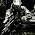VHDL coding tips and tricks: Fractional Clock Division (Dual modulus prescaler)

## Saturday, August 3, 2013

### Fractional Clock Division (Dual modulus prescaler)

In an earlier post I have talked about generating a lower frequency from a higher clock frequency, by means of integer division. The disadvantage of such a system is that the values of output frequencies are limited. Suppose the input frequency is 100 MHz, then the frequencies which can be generated are limited to (100/2) Mhz, (100/3) Mhz, (100/4) Mhz, (100/5) Mhz, (100/6) Mhz  etc..

In this post, I will take the case when you have to generate a frequency with a fractional division. For example how do we generate a (100/3.45) Mhz wave from a 100 MHz clock input? A method called Dual modulus prescaler is used for this.

Let me start with some basics:

Say,
f_in - the frequency of the input wave.
f_out - the frequency of the output wave.
f_acc - the channel spacing frequency(accuracy of the system).

Lets define,
M_real = f_in / f_out.

Choose an integer M such that,
M < M_real < (M+1)

Define an integer C ( total integer count ),
C = f_in / f_acc.

Define integers N and A,
N = floor (C / M)     ( C is always greater than M )
A = C mod M          ( A is always less than N )

Now how does it work? Basically there are two frequency dividers within the system. One divides the input frequency by M+1(lets call it output f1) and another divides it by M(lets call it output f2). There is also an integer counter which keeps counting from 1 to N. When the counter value is less than or equal to A, the f1 signal will be assigned to output. When the counter value is more than A, the f2 signal will be assigned. So in effect over a range of M*N+A clock cycles the effective frequency will be f_out.

There is a vhdl code available here. I have edited it a bit to make it generic. See the code below:

library ieee;
use ieee.std_logic_1164.all;

entity example_dual_mod is
port(
reset  : in  std_logic; -- Active-High Synchronous Reset
clock  : in  std_logic; -- Input Clock
output : out std_logic  -- Output Baud Clock
);
end example_dual_mod;

architecture implementation of example_dual_mod is

-- change these parameters for changing the output freq(in hz).
constant f_in : real := 10000000.0; -- input freq.
constant f_out : real := 115200.0; -- output freq.
constant f_acc : real := 100.0; --  channel spacing frequency

--NO need to edit the below lines.
constant Total_Count : integer := integer(f_in/f_acc);
constant N : integer := integer(f_in/f_out)+1; -- N should always be P+1
constant P : integer := N-1; -- P should always be N-1
constant C : integer := Total_Count/P; -- Sequence Length
constant B : integer := C-(Total_Count mod P); -- # of times to divide by P

signal seq_ctr       : integer range 0 to C-1; -- Sequence Counter
signal dual_mod_load : integer range 0 to N-1; -- Selected load value
signal dual_mod_ctr  : integer range 0 to N-1; -- Dual Modulus Counter
signal mux_select    : std_logic; -- Selects between N and P
signal term_count    : std_logic; -- Dual Modulus Terminal Count
signal divider       : std_logic; -- Output Divider

begin

-- This is the sequence counter. Count from C-1 downto 0. Enabled only
-- when term_count is active. If count is 0, then reload to C-1
pSeqCount: process(clock)
begin
if (rising_edge(clock)) then
if (reset = '1') then
seq_ctr <= 0;
else
if (term_count = '1') then
if (seq_ctr = 0) then
seq_ctr <= C-1;
else
seq_ctr <= seq_ctr - 1;
end if;
end if;
end if;
end if;
end process;

-- This is the comparison of the current sequence count to the value B
mux_select <= '1when (seq_ctr < B) else '0';

-- This statement implements the modulus selection multiplexer
dual_mod_load <= (P-1) when (mux_select = '1') else (N-1);

-- This is the dual-modulus counter. Count from dual_mod_load downto 0.
-- Counter auto reloads when terminal count is reached.
pDualModCount: process(clock)
begin
if (rising_edge(clock)) then
if (reset = '1') then
dual_mod_ctr <= 0;
else
if (term_count = '1') then
dual_mod_ctr <= dual_mod_load;
else
dual_mod_ctr <= dual_mod_ctr - 1;
end if;
end if;
end if;
end process;

-- Detect the terminal count condition
term_count <= '1when (dual_mod_ctr = 0) else '0';

-- The output divide-by-two counter
pDivider: process(clock)
begin
if (rising_edge(clock)) then
if (reset = '1') then
divider <= '0';
elsif (term_count = '1') then
divider <= not(divider);
end if;
end if;
end process;

-- Module Output
output <= divider;

end implementation;

I havent tested this code on any fpga board, but its synthesisable. It works in simulation, but in case there are any errors then please let me know by putting a comment down.

Note :- For more accuracy and jitter performances its better to use DCM.

#### 2 comments:

1.Hi There.
I have just tested this code on an Altera DE2 board, and for some reason it gives me half the frequency I select.
For example, if I set it to give me 115200 out of a 50MHz clock, it gives me 57600 instead.
Something may be off with the math I guess, I didn't have a chance to check though.
(PS.: the original code you poing to on the word document works fine, generating the 115200 out of a 10MHz clock - just thought your is more interesting because it is configurable)

Cheers,
Marcelo.

1.Hi, I guess that this thread is dead, but . . . .

This looked very interesting to me for my project too, but as Marcelo has said, the frequency is half of that selected. Could the originator please revisit this page and give us some idea on how to fix this please?
regards
Dave